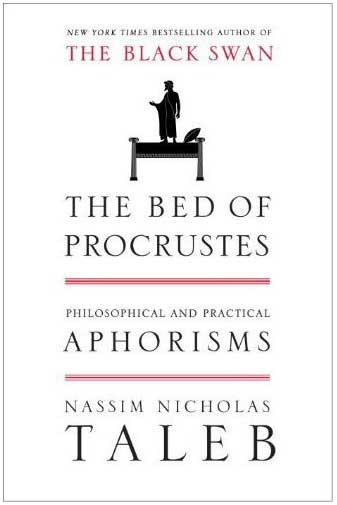## bias in estimating bracketed quantile contributions“Vilfredo Pareto noticed that 80% of the land in Italy belonged to 20% of the population, and vice-versa, thus both giving birth to the power law class of distributions and the popular saying 80/20.”

Yesterday, in “one of those” coincidences, I voluntarily dropped Nassim Taleb’s The Bed of Procrustes in a suburban café as my latest contribution to the book-crossing (or bXing!) concept and spotted a newly arXived paper by Taleb and Douadi. Paper which full title is “On the Biases and Variability in the Estimation of Concentration Using Bracketed Quantile Contributions” and which central idea is that estimating$\kappa_\alpha = \alpha\mathbb{E}[X|X>q_\alpha]\big/\mathbb{E}[X]$

(where qα is the α-level quantile of X) by the ratio$\sum_{i=1}^n \mathbb{I}_{X_i>\hat{q_\alpha}} X_i \big/ \sum_{i=1}^n X_i$

can be strongly biased. And that the fatter the tail (i.e. the lower the power β for a power law tail), the worse the bias. This is definitely correct, if not entirely surprising given that the estimating ratio involves a ratio of estimators, plus an estimator of qα. And that both numerator and denominator have finite variances when the power β is less than 2.  The paper contains a simulation experiment easily reproduced by the following R code

#biased estimator of kappa(.01)
alpha=.01 #tail
omalpha=1-alpha
T=10^4    #simulations
n=10^3    #sample size
beta=1.1  #Pareto parameter
moobeta=-1/beta

kap=rep(0,T)
for (t in 1:T){
sampl=runif(n)^moobeta
quanta=quantile(sampl,omalpha)
kap[t]=sum(sampl[sampl>quanta])/sum(sampl)
}


What is somewhat surprising though is that the paper deems it necessary to run T=10¹² simulations to assess the bias when this bias is already visible in the first digit of κα. Given that the simulation experiment goes as high as n=10⁸, this means the authors simulated 10²⁰ Pareto variables to exhibit a bias a few thousand replicas could have produced. Checking the numerators and denominators in the above collection of ratios also shows that they may take unbelievably large values.)

“…some theories are built based on claims of such increase’ in inequality, as in Piketti (2014), without taking into account the true nature of κ, and promulgating theories about the variation’ of inequality without reference to the stochasticity of the estimation—and the lack of consistency of κ across time and sub-units.”

The more relevant questions about this issue of estimating κα are, in my opinion, (a) why this quantity is of enough practical importance to consider its estimation and to seek estimators that would remain robust as the power β varies arbitrarily close to 1; (b) in which sense there is anything more to the phenomenon than the difficulty in estimating β itself;  and (c) what is the efficient asymptotic variance for estimating κα (since there is no particular reason to only consider the most natural estimator). Despite the above quote, that the paper constitutes  a major refutation of Piketty’s Capital in the Twenty-First Century is rather unlikely!

This site uses Akismet to reduce spam. Learn how your comment data is processed.